# Test: Angle Relations In Parallelogram

## 15 Questions MCQ Test Mathematics (Maths) Class 9 | Test: Angle Relations In Parallelogram

Description
This mock test of Test: Angle Relations In Parallelogram for Class 9 helps you for every Class 9 entrance exam. This contains 15 Multiple Choice Questions for Class 9 Test: Angle Relations In Parallelogram (mcq) to study with solutions a complete question bank. The solved questions answers in this Test: Angle Relations In Parallelogram quiz give you a good mix of easy questions and tough questions. Class 9 students definitely take this Test: Angle Relations In Parallelogram exercise for a better result in the exam. You can find other Test: Angle Relations In Parallelogram extra questions, long questions & short questions for Class 9 on EduRev as well by searching above.
QUESTION: 1

### The diagonals AC and BD of a parallelogram ABCD intersect each other at the point O. If ∠DAC = 32°, ∠AOB = 70°, then ∠DBC is equal to:

Solution:

<DBC = 38°

Step-by-step explanation: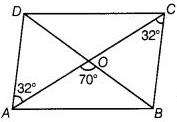Given:

ABCD is a parallelogram .

AC,BD diagonals intersecting at "O".

<DAC = 32°, <AOB = 70°

Proof:

i) AB//DC , AC is a transversal.

<DAC = <BCA = 32°

/* Alternate angles */

ii) In BCO , CO extended to A.

Sum of interior opposite angles = Exterior angle at O

=> <BCA + <OBC = <AOB

=> <BCA + <DBC = <AOB

=> 32° + <DBC = 70°

=> <DBC = 70°-32°

=> <DBC = 38°

QUESTION: 2

Solution:
QUESTION: 3

### In parallelogram ABCD, if ∠A = 2x + 15°, ∠B = 3x – 25°, then value of x is:

Solution:

2x+15+3x-25=180
[co interior angles]
5x-10=180
5x=190
x=38

QUESTION: 4

In a parallelogram the sum of two consecutive angles is

Solution:
QUESTION: 5

Bisectors of angles of a parallelogram form a

Solution: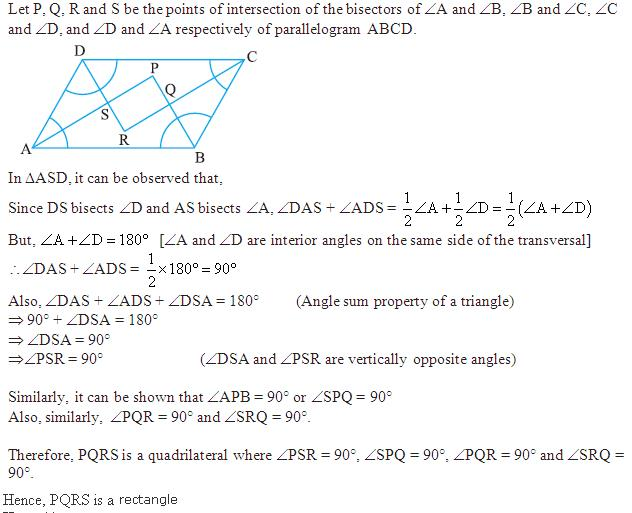QUESTION: 6

ABCD is a parallelogram in which ∠DAB = 75° and ∠DBC = 60°, the measure of ∠CDB and ∠ADB is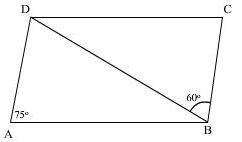Solution:
QUESTION: 7

In a parallelogram, if diagonal PR bisects the ∠P, then it also bisects _____.

Solution:
QUESTION: 8

ABCD is a parallelogram in which the bisectors of angles ∠A and ∠B intersect at point P then ∠APB is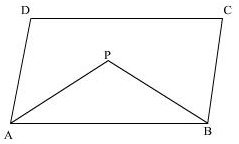Solution:
QUESTION: 9

One angle of a parallelogram is 30° less than twice the smallest angle then the measure of each angle of the parallelogram is

Solution:
QUESTION: 10

Perimeter of a parallelogram is 22 cm. If the longer side, measures 6.5 cm, the measure of the shorter side will be

Solution:
QUESTION: 11

If PQRS is a parallelogram, then ∠Q – ∠S is equal to:

Solution:
QUESTION: 12

The diagonals of a rectangle PQRS intersects at O. If ∠QOR = 44°, ∠OPS =?

Solution:
QUESTION: 13

In the given figure, PQRS is a parallelogram and ∠R is 100° then ∠Q is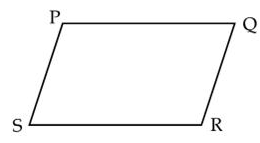Solution:
QUESTION: 14

In a parallelogram ABCD, if ∠A = 75°, then ∠B = ?

Solution:
QUESTION: 15

AN and CP are perpendiculars to the diagonal BD of a parallelogram ABCD. Choose the correct option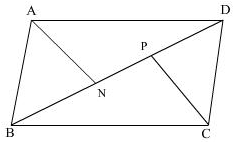Solution: